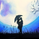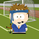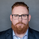‎17324‎ مشاهدة
17324
This strategy is based off of Chris Moody's Vix Fix Indicator. I simply used his indicator and added some rules around it, specifically on entry and exits.

Rules:
• Enter upon a filtered or aggressive entry
• If there are multiple entry signals, allow pyramiding
• Exit when there is Stochastic RSI crossover above 80

This works great on a number of stocks. I am keeping a list of stocks with decent Profit Factors and clean equity curves here.

Possible ways to use this:
• Modify this script and setup alerts around the various entries
• Use as is with different stocks or currency pairs
• Modify entry / exit points to make it more profitable for even more symbols and currencies
```//@version=2
strategy("Vix FIX / StochRSI Strategy", pyramiding=9, initial_capital=10000, default_qty_type=strategy.percent_of_equity, default_qty_value=3, overlay=false)

///////////// Stochastic Slow
Stochlength = input(14, minval=1, title="lookback length of Stochastic")
StochOverBought = input(80, title="Stochastic overbought condition")
StochOverSold = input(20, title="Stochastic oversold condition")
smoothK = input(3, title="smoothing of Stochastic %K ")
smoothD = input(3, title="moving average of Stochastic %K")
k = sma(stoch(close, high, low, Stochlength), smoothK)
d = sma(k, smoothD)

///////////// RSI
RSIlength = input( 14, minval=1 , title="lookback length of RSI")
RSIOverBought = input( 70  , title="RSI overbought condition")
RSIOverSold = input( 30  , title="RSI oversold condition")
RSIprice = close
vrsi = rsi(RSIprice, RSIlength)

///////////// Double strategy: RSI strategy + Stochastic strategy

pd = input(22, title="LookBack Period Standard Deviation High")
bbl = input(20, title="Bolinger Band Length")
mult = input(2.0    , minval=1, maxval=5, title="Bollinger Band Standard Devaition Up")
lb = input(50  , title="Look Back Period Percentile High")
ph = input(.85, title="Highest Percentile - 0.90=90%, 0.95=95%, 0.99=99%")
new = input(false, title="-------Text Plots Below Use Original Criteria-------" )
sbc = input(false, title="Show Text Plot if WVF WAS True and IS Now False")
sbcc = input(false, title="Show Text Plot if WVF IS True")
new2 = input(false, title="-------Text Plots Below Use FILTERED Criteria-------" )
sbcFilt = input(true, title="Show Text Plot For Filtered Entry")
sbcAggr = input(true, title="Show Text Plot For AGGRESSIVE Filtered Entry")
ltLB = input(40, minval=25, maxval=99, title="Long-Term Look Back Current Bar Has To Close Below This Value OR Medium Term--Default=40")
mtLB = input(14, minval=10, maxval=20, title="Medium-Term Look Back Current Bar Has To Close Below This Value OR Long Term--Default=14")
str = input(3, minval=1, maxval=9, title="Entry Price Action Strength--Close > X Bars Back---Default=3")
//Alerts Instructions and Options Below...Inputs Tab
new4 = input(false, title="-------------------------Turn On/Off ALERTS Below---------------------" )
new5 = input(false, title="----To Activate Alerts You HAVE To Check The Boxes Below For Any Alert Criteria You Want----")
sa1 = input(false, title="Show Alert WVF = True?")
sa2 = input(false, title="Show Alert WVF Was True Now False?")
sa3 = input(false, title="Show Alert WVF Filtered?")
sa4 = input(false, title="Show Alert WVF AGGRESSIVE Filter?")

//Williams Vix Fix Formula
wvf = ((highest(close, pd)-low)/(highest(close, pd)))*100
sDev = mult * stdev(wvf, bbl)
midLine = sma(wvf, bbl)
lowerBand = midLine - sDev
upperBand = midLine + sDev
rangeHigh = (highest(wvf, lb)) * ph

//Filtered Bar Criteria
upRange = low > low and close > high
upRange_Aggr = close > close and close > open
//Filtered Criteria
filtered = ((wvf >= upperBand or wvf >= rangeHigh) and (wvf < upperBand and wvf < rangeHigh))
filtered_Aggr = (wvf >= upperBand or wvf >= rangeHigh) and not (wvf < upperBand and wvf < rangeHigh)

alert1 = wvf >= upperBand or wvf >= rangeHigh ? 1 : 0
alert2 = (wvf >= upperBand or wvf >= rangeHigh) and (wvf < upperBand and wvf < rangeHigh) ? 1 : 0
alert3 = upRange and close > close[str] and (close < close[ltLB] or close < close[mtLB]) and filtered ? 1 : 0
alert4 = upRange_Aggr and close > close[str] and (close < close[ltLB] or close < close[mtLB]) and filtered_Aggr ? 1 : 0

//Coloring Criteria of Williams Vix Fix
col = wvf >= upperBand or wvf >= rangeHigh ? lime : gray

isOverBought = (crossover(k,d) and k > StochOverBought) ? 1 : 0
isOverBoughtv2 = k > StochOverBought ? 1 : 0

plot(isOverBought, "Overbought / Crossover", style=line, color=red)

strategy.entry("Long", strategy.long)

if (isOverBought)
strategy.close("Long")

```

## التعليقاتthanks for sharing such a great thing!
by the way, what period you use to trade with that strategy? did you try to mix VIX signals from different periods to make better Winning Percentage?
ردHi, in the pine code you define vrsi, alert1 and alert2, but this variables are not used later on. Why are they defined, but not used?
ردmarco.dillenburg
@marco.dillenburg, I'm not entirely sure - was probably testing something out
ردtimj
@timj, Hi, you could use vrsi to generate a RSI based signal additionaly:
isLongEntry = close > ma200 and close < ma5 and vrsi < 10
isLongExit = close > ma200 and close < ma5 and high > ma5 and ((close > ma200 and close < ma5 and vrsi < 10) or (close > ma200 and close < ma5 and vrsi < 10) or (close > ma200 and close < ma5 and vrsi < 10) or (close > ma200 and close < ma5 and vrsi < 10) )
isShortEntry = close < ma200 and close > ma5 and vrsi > 90
isShortExit = close < ma200 and close > ma5 and low < ma5 and ((close < ma200 and close > ma5 and vrsi > 90) or (close < ma200 and close > ma5 and vrsi > 90) or (close < ma200 and close > ma5 and vrsi > 90) or (close < ma200 and close > ma5 and vrsi > 90) )

The alerts could be used only for visualization purpose when the VIX fix is true or not.
رد